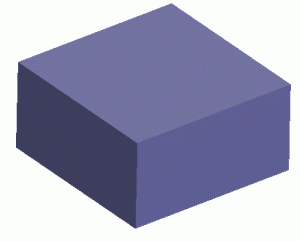# Water Volume Calculation Formula of a Rectangle

Knowing how much water your tanks hold is very important in aquaponics. Here are the formulas for calculating water volume in a rectangle grow beds.### How to Calculate the Volume of Water in a Rectangle

I am using a sheet of Firestone 10 X 15 Liner-45mil EPDM. I cut that in half for 2 sheets, and then build rectangle troughs or grow beds out of them. The measurements are approximately 30″ wide x 150″ long x 12″ deep. I default to these numbers to show you the example, but you can enter any numbers you want for your specifics.

 First, we need to get the cubic inches (wide x long x deep): Width (inches) Length (inches) Depth (inches) Cubic Inches Now we convert to cubic feet (cubic inches x 0.000578704) Cubic Feet To calculate gallons, we multiply cubic feet by 7.47: Gallons (Water only) Gallons (Water + Grow Media) To figure out how much this weighs (and if your subfloor can support the weight), we multiply 8.34 (freshwater weight of 1 gallon) x how many gallons: Weight (only the weight of the water) Note that if you are using this in grow beds, you will likely only get half of the water you plan for into the grow bed, the rest is filled with media (like gravel or hydroton).

Previous post:

Next post: